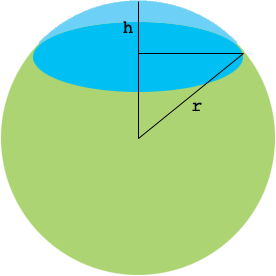SEARCH HOMEMath Central Quandaries & QueriesQuestion from Shanto: In a jar of water, we kept a sphere with radius r. Then 75% of its volume went under water. Find out the distance of the top of the sphere from the surface of the water.Hi Shanto,

The key here is to know an expression for the volume of a spherical cap. In my diagram I have shaded the region below the water surface green. What you need is an expression for the volume of the blue region in terms of $r$ and $h.$If you know some calculus you can find this volume using a volume of revolution technique. You can also look it up on the web.

PennyMath Central is supported by the University of Regina and The Pacific Institute for the Mathematical Sciences.